# Multiple Linear Regression using R

Linear Regression:
It is the basic and commonly used used type for predictive analysis.It is a statistical approach for modelling relationship between a dependent variable and a given set of independent variables.

These are of two types:

1. Simple linear Regression
2. Multiple Linear Regression

Let’s Discuss about Multiple Linear Regression using R.

Multiple Linear Regression :
It is the most common form of Linear Regression. Multiple Linear Regression basically describes how a single response variable Y depends linearly on a number of predictor variables.

The basic examples where Multiple Regression can be used are as follows:

1. The selling price of a house can depend on the desirability of the location, the number of bedrooms, the number of bathrooms, the year the house was built, the square footage of the lot and a number of other factors.
2. The height of a child can depend on the height of the mother, the height of the father, nutrition, and environmental factors.

Estimation of the Model Parameters
Consider a multiple linear Regression model with k independent predictor variable x1, x2……, xk and one response variable y.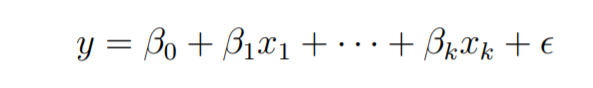Suppose we have n observation on the k+1 variables and the variable of n should be greater than k.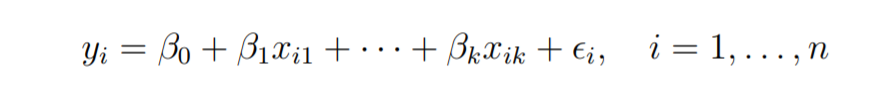The basic goal in least-squares regression is to fit a hyper-plane into (k + 1)-dimensional space that minimizes the sum of squared residuals.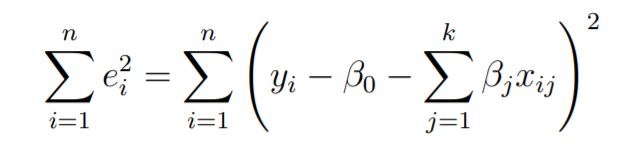Before taking the derivative with respect to the model parameters set them equal to zero and derive the least-squares normal equations that the parameters would have to fulfill.
These equations are formulated with the help of vectors and matrices.
Let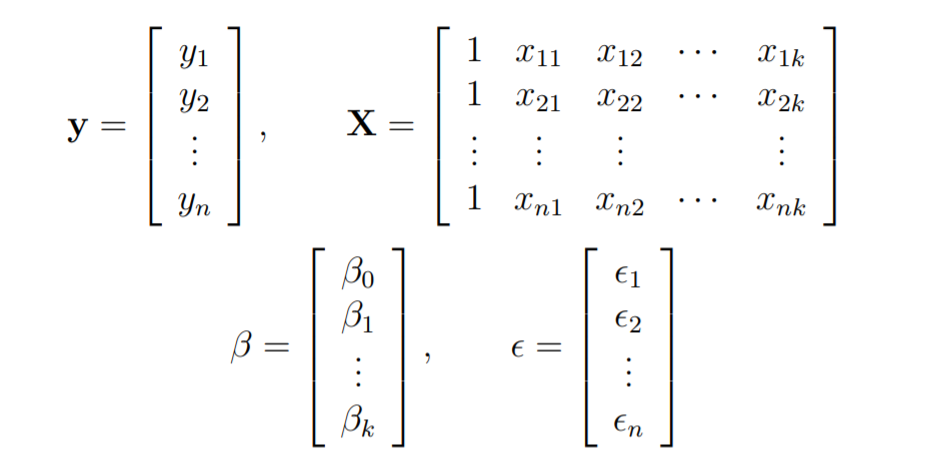The linear Regression model is written in the form as follows: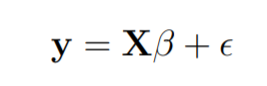In linear regression the least square parameters estimates b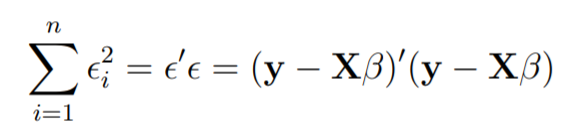Imagine the columns of X to be fixed, they are the data for a specific problem, and say b to be variable. We want to find the “best” b in the sense that the sum of squared residuals is minimized.
The smallest that the sum of squares could be is zero.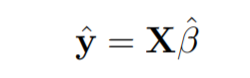Here y is the estimated response vector.

Following R code is used to implement Multiple Linear Regression on following dataset.

dataset looks like this: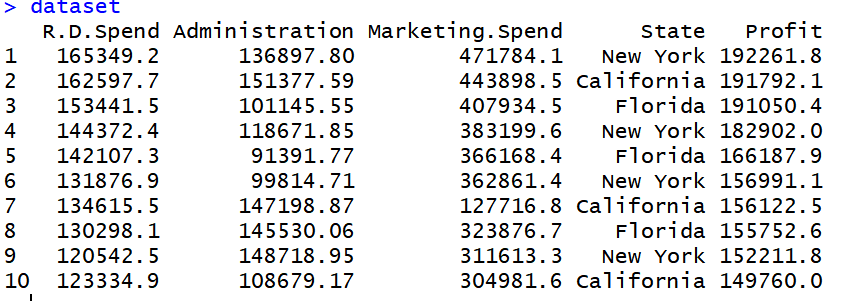`# Multiple Linear Regression ` ` ` `# Importing the dataset ` `dataset = ``read.csv``(``'data2.csv'``) ` ` ` `# Encoding categorical data ` `dataset\$State = ``factor``(dataset\$State, ` `                       ``levels = ``c``(``'New York'``, ``'California'``, ``'Florida'``), ` `                       ``labels = ``c``(1, 2, 3)) ` `dataset\$State `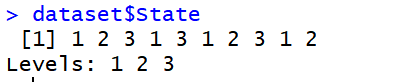`# Splitting the dataset into the Training set and Test set ` `# install.packages('caTools') ` `library``(caTools) ` `set.seed``(123) ` `split = ``sample.split``(dataset\$Profit, SplitRatio = 0.8) ` `training_set = ``subset``(dataset, split == ``TRUE``) ` `test_set = ``subset``(dataset, split == ``FALSE``) ` ` ` `# Feature Scaling ` `# training_set = scale(training_set) ` `# test_set = scale(test_set) ` ` ` `# Fitting Multiple Linear Regression to the Training set ` `regressor = ``lm``(formula = Profit ~ ., ` `               ``data = training_set) ` ` ` `# Predicting the Test set results ` `y_pred = ``predict``(regressor, newdata = test_set) `

Output: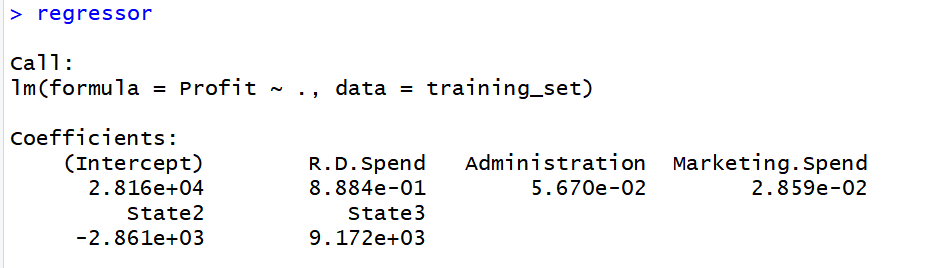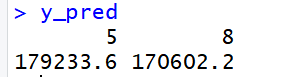# Special 95% discount

## 2000+ Applied Machine Learning & Data Science Recipes

### Portfolio Projects for Aspiring Data Scientists: Tabular Text & Image Data Analytics as well as Time Series Forecasting in Python & R## Two Machine Learning Fields

There are two sides to machine learning:

• Practical Machine Learning:This is about querying databases, cleaning data, writing scripts to transform data and gluing algorithm and libraries together and writing custom code to squeeze reliable answers from data to satisfy difficult and ill defined questions. It’s the mess of reality.
• Theoretical Machine Learning: This is about math and abstraction and idealized scenarios and limits and beauty and informing what is possible. It is a whole lot neater and cleaner and removed from the mess of reality.

Data Science Resources: Data Science Recipes and Applied Machine Learning Recipes

Introduction to Applied Machine Learning & Data Science for Beginners, Business Analysts, Students, Researchers and Freelancers with Python & R Codes @ Western Australian Center for Applied Machine Learning & Data Science (WACAMLDS) !!!

Latest end-to-end Learn by Coding Recipes in Project-Based Learning:

Applied Statistics with R for Beginners and Business Professionals

Data Science and Machine Learning Projects in Python: Tabular Data Analytics

Data Science and Machine Learning Projects in R: Tabular Data Analytics

Python Machine Learning & Data Science Recipes: Learn by Coding

R Machine Learning & Data Science Recipes: Learn by Coding

Comparing Different Machine Learning Algorithms in Python for Classification (FREE)

`Disclaimer: The information and code presented within this recipe/tutorial is only for educational and coaching purposes for beginners and developers. Anyone can practice and apply the recipe/tutorial presented here, but the reader is taking full responsibility for his/her actions. The author (content curator) of this recipe (code / program) has made every effort to ensure the accuracy of the information was correct at time of publication. The author (content curator) does not assume and hereby disclaims any liability to any party for any loss, damage, or disruption caused by errors or omissions, whether such errors or omissions result from accident, negligence, or any other cause. The information presented here could also be found in public knowledge domains.  `